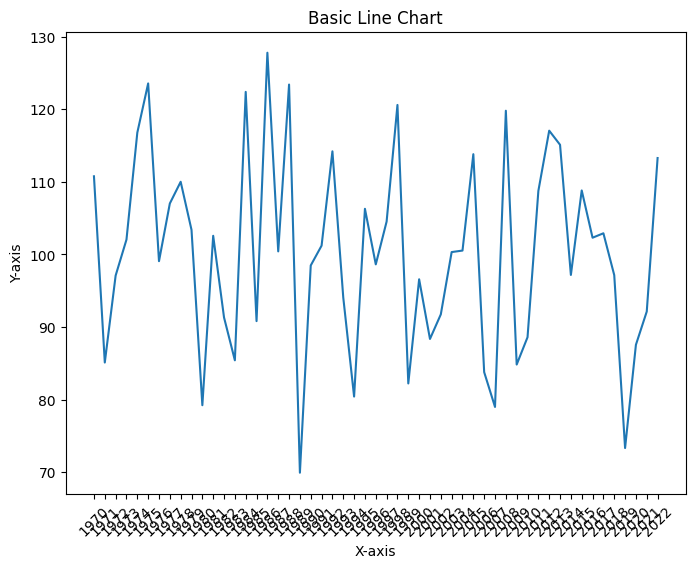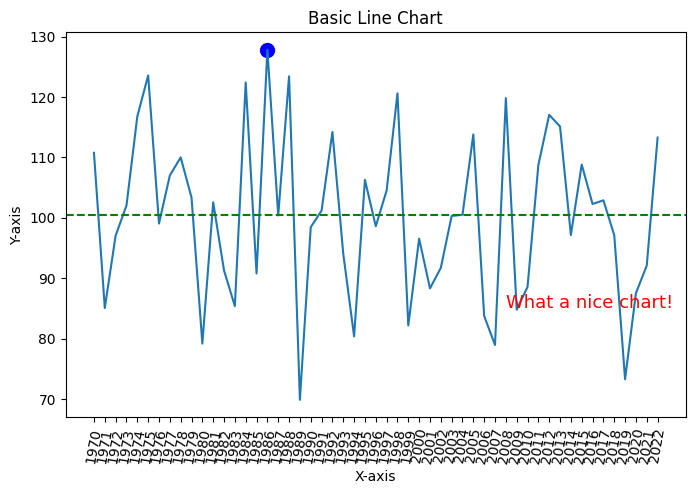### Principles

A line chart is a type of visual representation that uses a series of points connected by lines to show how data changes over time. It's like connecting the dots on a graph to reveal trends and patterns. Line charts are useful for tracking things that happen over a period, such as temperature changes, stock prices, or your savings in a piggy bank. They help us quickly see if something goes up, down, or stays steady as time passes, making it easier to understand how things are changing and make informed decisions.

### Annotations

Annotations are an essential tool for enhancing the clarity and insight of line charts. They allow you to highlight specific data points, events, or trends on the chart. In this tutorial, we will walk through the process of creating line charts with annotations using the Matplotlib library in Python.

## Libraries

First, you need to install the following librairies:

• matplotlib is used for plot creating the charts
• `numpy` is used to generate some data
• `pandas` is used to put the data into a dataframe
``````# Libraries
import matplotlib.pyplot as plt
import numpy as np
import pandas as pd``````

## Dataset

Line chart are generally used to represent variations over time, but they don't have to be. For example, you can look at connected scatter plots.

In our case, however, we will generate dates. To do this, we will simply use a `for` loop and generate annual data, from 1970 to 2022.

Then we generate random values using `np.random.normal()` function from `numpy`.

``````# Init an empty list that will stores the dates
dates = []

# Iterates over our range and add the value to the list
for date in range(1970,2023):
dates.append(str(date))

# Generate a random variable
sample_size = 2023-1970
variable = np.random.normal(100, # mean
15, # standard deviation
sample_size, # sample size
)

df = pd.DataFrame({'date': dates,
'value': variable})``````

## Basic line chart

The following code displays a simple line chart, with a title and an axis name, thanks to the `plot()` function.

``````# Set the fig size
plt.figure(figsize=(8, 6))

# Create the line chart
plt.plot(df['date'],
df['value'])

# Add labels and a title
plt.xlabel('X-axis')
plt.ylabel('Y-axis')
plt.title('Basic Line Chart')

# Rotate x-axis labels by 45 degrees for better visibility
plt.xticks(rotation=45)

# Display the chart
plt.show()``````## Line chart with annotations

In the following code, we will add 3 different annotations:

• A reference line: the median (calculated thanks to the `median()` method from `pandas`)
• A circle: the highest value (calculated thanks to `idxmax()` method from `pandas` that returns us the index of the highest value)
• A text: just a fun comment

The `iloc()` function is a powerful tool in the `pandas` library that allows you to access data in a DataFrame using integer-based indexing. It stands for "integer location" and provides a way to select specific rows and columns based on their numerical positions, rather than labels.

``````import matplotlib.pyplot as plt

# Set the fig size
plt.figure(figsize=(8, 5))

# Create the line chart
plt.plot(df['date'],
df['value'])

# Add labels and a title
plt.xlabel('X-axis')
plt.ylabel('Y-axis')
plt.title('Basic Line Chart')

# Rotate x-axis labels by 45 degrees for better visibility
plt.xticks(rotation=80)

plt.text(df['date'].iloc, # x-axis position
df['value'].iloc, # y-axis position
'What a nice chart!', # text displayed
fontsize=13,
color='red')

# Find the index of the highest value
highest_index = df['value'].idxmax()

# Add a circle annotation at the highest value
plt.scatter(df['date'].iloc[highest_index],
df['value'].iloc[highest_index],
color='blue',
marker='o', # Specify that we want a circle
s=100, # Size
)

# Calculate the median value
median_value = df['value'].median()

# Add a reference line at the median value
plt.axhline(y=median_value, color='green', linestyle='--', label='Reference Line (Median)')

# Display the chart
plt.show()``````## Going further

This article explains how to create a line chart with annotation with Matplotlib.

For more examples of how to create or customize your line charts with Python, see the line chart section. You may also be interested in adding an image/logo to your charts.

## Contact & Edit

👋 This document is a work by Yan Holtz. You can contribute on github, send me a feedback on twitter or subscribe to the newsletter to know when new examples are published! 🔥